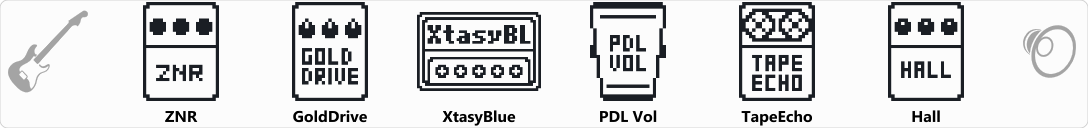# bogner extasy for amp

Discussion in 'Zoom G3n/G3Xn/G5n' started by Davide, Sep 1, 2018.

1. bogner extasy for ampDevice: Zoom G5n
Firmware: 3.00

Name on device: Xtasy Amp
Optimized for: Guitar Amp

Effects chain:for Amp

Effect: "ZNR" (Dynamics), active - "yes"
"Detect" = EFXIN
"Depth" = 100
"Threshold" = 30
"Decay" = 0

Effect: "GoldDrive" (Overdrive / Distortion), active - "yes"
"Gain" = 48
"Bass" = 65
"Treble" = 56
"Volume" = 43

Effect: "XtasyBlue" (Amp simulator), active - "yes"
"Bass" = 50
"Middle" = 50
"Treble" = 50
"Presence" = 40
"STRCT" = HI
"Gain" = 60
"Volume" = 42
"SOLO" = 3

Effect: "PDL Vol" (Pedal), active - "yes"
"Volume" = 100
"Min" = 0
"Max" = 100
"Curve" = B

Effect: "TapeEcho" (Delay), active - "yes"
"Time" = Quarter
"F.B." = 64
"Mix" = 49
"Tail" = On

Effect: "Hall" (Reverb), active - "yes"
"PreD" = 49
"Decay" = 10
"Mix" = 10
"Tail" = Off

Patch Volume: 100